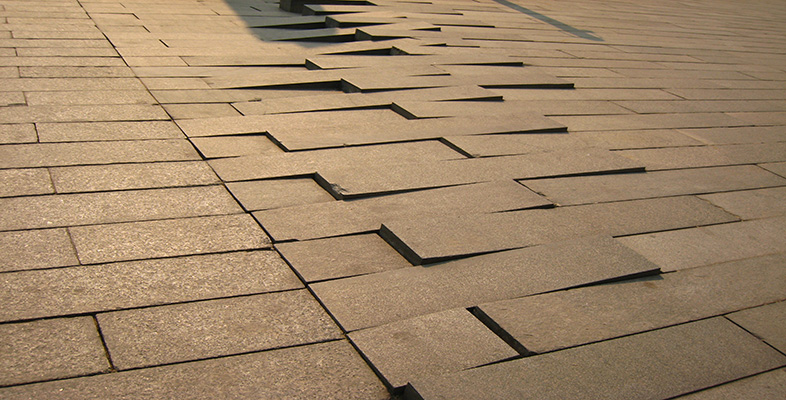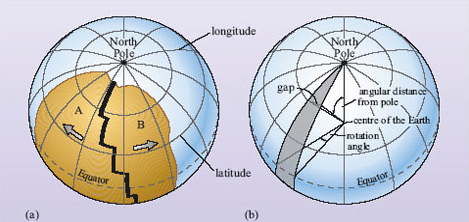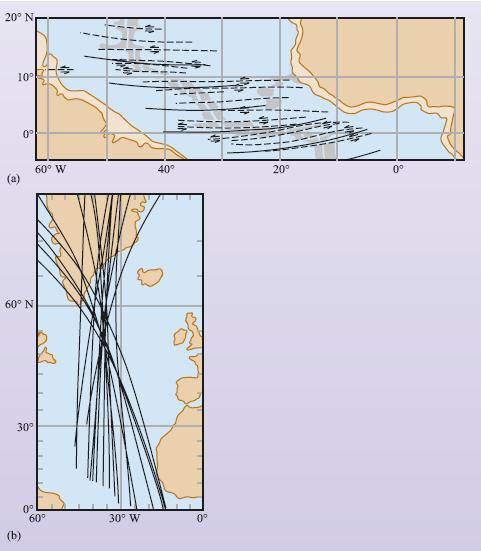Science, Maths & Technology

### Become an OU studentPlate tectonics

Start this free course now. Just create an account and sign in. Enrol and complete the course for a free statement of participation or digital badge if available.

# 4.3 Plate motion on a spherical Earth

Earth's tectonic plates are continuously in motion with respect to each other, and together they form the closed surface of a sphere (i.e. the Earth's surface). Understanding the movement of plates, therefore, requires a geometrical analysis of motions over a spherical surface. This is described in the Euler (pronounced 'oiler') geometrical theorem, which shows that every displacement of a plate from one position to another on the Earth's surface can be regarded as a simple rotation of that plate about a suitably chosen axis, known as an Euler pole or pole of rotation, which passes through the centre of the Earth.

Plate movement on a spherical Earth is illustrated in Figure 26. Two plates, A and B, are separating at a constructive plate boundary and rotating about a pole of rotation (which in this case is the North Pole). The constructive plate boundaries lie along lines of longitude, whereas the transform faults are parallel to lines of latitude. Lines of longitude are great circles with centres that coincide with the centre of the Earth; lines of latitude, which are at right angles to lines of longitude, are small circles with centres displaced from the Earth's centre. When the plates separate, the gap between them increases but, quite obviously, they separate a smaller amount close to the North Pole than at the Equator.Figure 26 (a) The geometry of a constructive boundary in which two plates are separating. (b) The geometrical relationships that may be used to describe plate movement. Note that whilst the rotation angle remains the same along the length of the split, the width of the gap increases with angular distance from the pole of rotation (in this case the North Pole).

## Question 21

What effect does this have on local full and half spreading rates along the length of the constructive plate boundary?

Spreading rates measured close to the pole of rotation will be small while those further away will be much greater.

This aspect of spherical geometry explains why relative plate motions expressed simply in terms of mm y−1 vary along the length of a plate margin. Needless to say, poles of rotation are not all located at the geographical poles but can fall anywhere on the Earth's surface. For example, the Mid-Atlantic Ridge has variable spreading rates along its length, with values of 37-40 mm y−1 in the South Atlantic, to lower values of 23-26 mm y−1 in the North Atlantic (Figure 8).

## Question 22

What do these variations in spreading rate tell you about the location of the pole of rotation of the South American Plate?